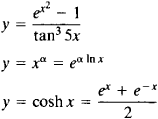# Elementary Function

(redirected from Elementary function (disambiguation))

## elementary function

[‚el·ə′men·trē ′fəŋk·shən]
(mathematics)
Any function which can be formed from algebraic functions and the exponential, logarithmic, and trigonometric functions by a finite number of operations consisting of addition, subtraction, multiplication, division, and composition of functions.
McGraw-Hill Dictionary of Scientific & Technical Terms, 6E, Copyright © 2003 by The McGraw-Hill Companies, Inc.
The following article is from The Great Soviet Encyclopedia (1979). It might be outdated or ideologically biased.

## Elementary Function

any function in the class consisting of polynomials, rational functions, exponential functions, logarithmic functions, trigonometric functions, and inverse trigonometric functions. The class of elementary functions also includes functions obtained from those listed above through the performance of the four arithmetic operations and composition (the formation of a composite function) a finite number of times; examples areThe class of elementary functions has been best studied, and is most often encountered, in applications of mathematics. Many practical problems, however, lead to the consideration of functions that are not elementary functions, such as cylindrical functions. The derivative of an elementary function is also an elementary function. The indefinite integral of an elementary function is not always expressed in terms of an elementary function. Nonelementary functions are often represented in terms of elementary functions through the use of, for example, infinite series, infinite products, and infinite integrals.

The Great Soviet Encyclopedia, 3rd Edition (1970-1979). © 2010 The Gale Group, Inc. All rights reserved.
Site: Follow: Share:
Open / Close